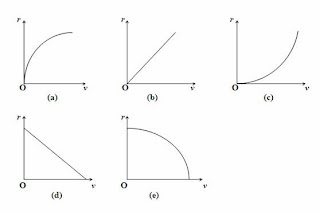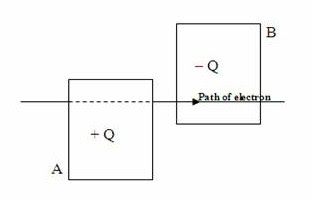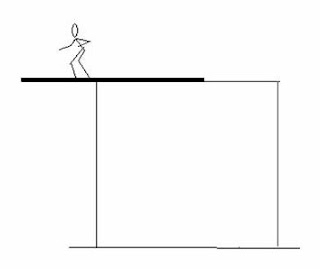## Pages

`“Life is like riding a bicycle.  To keep your balance you must keep moving.”–Albert Einstein`

## Friday, February 24, 2012

### AP Physics B & C - Multiple Choice Practice Questions on Magnetic Fields

“The best way to find yourself is to lose yourself in the service of others."
– Mahatma Gandhi

Questions on magnetic fields due to current carrying wires and the magnetic force on moving charges and current carrying wires are generally interesting. Such questions have been discussed on many occasions on this site. You can access them by clicking on the label ‘magnetic field’ below this post. Note that the percentage goal from this section for AP Physics C Electricity and Magnetism Exam is 20% while that for AP Physics B Exam is 4% (for the May 2012 Exam)
Today we will discuss a few more multiple choice practice questions in this section
(1) An electron moves with speed v in a direction perpendicular to a constant magnetic field B. Which one of the following graphs best represents the radius r of the path of the electron as a function of its speedThe radius r of the circular path followed by the electron in the perpendicular magnetic field B is given by
r = mv/qB where m is the mass of the electron and q is its charge.
[The above expression is obtained by equating the centripetal force to the magnetic force on the electron:
mv2/r = qvB]
In a constant magnetic field the radius r is directly proportional to the speed v and the graph shown in option (b) is the answer.(2) Two vertical metal plates A and B are arranged in an evacuated chamber with their planes parallel to each other and they carry charges + Q and – Q as indicated in the adjoining figure. A uniform magnetic field B exists in the region between the plates. An electron entering with horizontal velocity ‘v’ rightwards in the central region between the plates is found to proceed undeviated throughout the region. What is the direction of the magnetic field? (Ignore the effect of gravity)
(a) horizontally leftwards
(b) horizontally towards plate A
(c) horizontally towards plate B
(d) vertically downwards
(e) vertically upwards
Since the electron is negatively charged, the electric field in the region between the plates will exert a horizontal force on the electron. This force is directed towards the positive plate A. Since the electron proceeds undeviated throughout the region between the plates, the magnetic force on the electron must be equal and opposite to the electric force. This means that the magnetic force on the electron is directed horizontally towards the plate B
The rightward moving electron is equivalent to a leftward current. Therefore, on applying Fleming’s left hand rule, we find that the magnetic field must act vertically upwards.
[To apply Fleming’s left hand rule, hold the fore finger, middle finger and thumb of your left hand along mutually perpendicular directions such that the middle finger points along the direction of the current (horizontally leftwards in the present case) and the thumb points along the direction of the force (horizontally towards plate B in the present case). Your fore finger will then point vertically upwards].
(3) If the magnitude of the electric field in the above question is E and the mass and speed of the electron are respectively m and v, what is the magnitude of the magnetic field in between the plates?
(a) E/v
(b) Ev/m
(c) E/mv
(d) v/E
(e) E
Since the electron proceeds undeviated under the action of crossed electric and magnetic fields, we can equate the magnitudes of the magnetic and electric forces. Therefore, we have
Bev = Ee
This gives B = E/v.
[The mass of the electron given in the question just serves the purpose of a distraction]

(4) A charged particle entering at right angles to a uniform magnetic field has its trajectory as shown in the figure, during a small time interval. The magnetic field is directed normally into the plane of the trajectory and the speed of the particle is decreasing continuously. Which one among the following statements is correct?
(a) The particle is negatively charged and is moving in the clockwise sense
(b) The particle is negatively charged and is moving in the anticlockwise sense
(c) The particle is positively charged and is moving in the clockwise sense
(d) The particle is positively charged and is moving in the clockwise sense
(e) Nothing can be conclusively mentioned about the motion of the particle since the nature of its charge is not given
The particle must be moving in the clockwise sense since the radius of its initial path has to be greater because of the greater initial speed.
[We have radius r = mv/qB so that r α v. Qualitatively speaking, a particle with larger velocity cannot be easily affected by a force].
On applying Fleming’s left hand rule (motor rule) it is seen that the particle is negatively charged.
[When you hold the fore finger of your left hand along the direction of the magnetic field (normally into the plane of the figure) and the middle finger along the initial direction of the particle, you will find that the thumb is pointing radially outwards and not inwards as required for the curved trajectory given in the figure. This indicates that the direction of the conventional current is opposite to the direction of motion of the particle. Therefore the particle must be negatively charged.
(5) A straight wire of length 0.2 m is oriented along the z-axis. It carries a current of 5 A flowing along the positive z-direction. The magnetic force acting on this wire when it is placed in a magnetic field of flux density 0.4 j – 0.5 k tesla is (i, j and k are unit vectors along the x, y and z-directions respectively)
(a) – 0.4 j N
(b) 0.4 k N
(c) 0.9 i N
(d) – 0.4 i N
(e) 0.9 i N
The magnetic force F on the wire is given by
F = I L×B = 5[(0.2 k)×(0.4 j – 0.5 k)] = – 0.4 i newton.

[The magnetic force on the wire acts along the negative x-direction].

## Saturday, February 11, 2012

### AP Physics B & C - Multiple Choice Practice Questions on Circular Motion and Rotation

"I know not with what weapons World War III will be fought, but World War IV will be fought with sticks and stones."

Albert Einstein

Questions on circular motion and rotation were discussed on many occasions on this site. You can access all those questions by clicking on the label ‘circular motion and rotation’ below this post. Or, you may try a search for circular motion and rotation using the search box provided on this page.

Today we will discuss a few more multiple choice practice questions in this section.

The following questions will be useful for AP Physics B as well as AP Physics C aspirants:(1) A 7-metre long uniform iron girder of mass 300 kg is placed on the horizontal top of a building with 3 m extending over the edge of the building. A fireman weighing 75 kg starts walking towards the protruding end of the girder (Fig.). What is the maximum distance (from the edge of the building) upto which he can walk safely?

(a) 2 m

(b) 2.2 m

(c) 2.4 m

(d) 2.5 m

(e) 2.6 m

When the fireman moves just beyond the maximum safe distance, the girder starts rotating about the ege of the building. If the maximum safe istance is d, we have

75×d = 300×0.5

[We have equated the magnitude of the moment of the weight of the fireman (which is the deflecting torque due to the fireman’s weight) to the magnitude of the moment of the weight of the girder (which is the restoring torque due to the weight of the girder): 75g×d = 300g×0.5]

This gives d = 2 m.(2) A uniform metre stick of mass 0.2 kg can be balanced horizontally on a knife edge at the 40 cm mark, when a stone is suspended (using a string of negligible mass) from the 20 cm mark. The mass of the stone is

(a) 0.5 kg

(b) 0.3 kg

(c) 0.2 kg

(d) 0.1 kg

(e) 0.05 kg

The weight of the metre stick acts through its centre of gravity located at its middle (the 50 cm mark). Since metre stick is in equilibrium, the moment of the weight of the metre stick about the knife edge is equal and opposite to the moment of the weight of the stone about the knife edge. Therefore we have (on equating the magnitudes of the moments)

0.2g×0.1 = Mg×0.2 where M is the mass of the stone.

[Note that the distance of the knife edge from the centre of gravity of the metre stick is 0.1 m where as the distance from the line of action of the weight of the stone is 0.2 m].

(3) A square plate ABCD of side ‘a and mass ‘m’ is suspended so that it can rotate about a horizontal axis passing through A and perpendicular to its plane. To rotate the plate, a horizontal force of magnitude F is applied to the plate at the corner C as shown in the figure. What is the magnitude of the torque produced by this force?

(a) aF

(b) maF

(c) (√2)aF

(d) aF/√2

(e) 2maF

The distance of the axis of rotation from the line of action of the force is √(a2+a2) = (√2)a. Therefore, the magnitude of the torque is (√2)aF.

[The mass of the plate (m) in the question just serves the purpose of distracting you!]

The following questions are meant for AP Physics C aspirants:

(4) A disc (of mass M and radius R) and a ring (of mass M and radius 2R) are released from rest from the top of an inclined plane. If they roll down the plane without slipping, which one of the following statements is correct?

(a) The ring will reach the bottom of the incline earlier

(b) They will reach the bottom of the incline with the same speed

(c) The ring will reach the bottom of the incline with twice the speed of the disc

(d) They will have the same acceleration down the incline

(e) They will reach the bottom of the incline with the same kinetic energy

Since the disc and the ring have the same mass and both have fallen down through the same height, they have lost the same amount of gravitational potential energy. Therefore, they have gained the same amount of kinetic energy so that the correct option is (e). Since the above question is a single correct answer type multiple choice question, you can easily arrive at the above answer.

[Let us consider the remaining options in the question (in view of the benefits you will have in certain situations).

The linear acceleration ‘a of a body rolling down an inclined plane of angle θ is given by

a = g sinθ/[1+(k2/R2)] where g is the gravitational acceleration, k is the radius of gyration about the axis of rolling and R is the radius of the rolling body.

In the case of a ring k2/R2 = 1 where as in the case of a disc k2/R2 = ½

(Remember that the moment of inertia of a body is k2 times the mass of the body).

The acceleration of the ring is less than that of the disc. In fact a ring (and a pipe) has the least acceleration while rolling down an incline. Options (a), (b), (c) and (d) are therefore wrong].(5) A fly wheel has radius R and its axle has radius R/10. The ends of the axle are mounted on ball bearings so that the wheel can rotate without appreciable friction at the bearings. Strings are wound round the rim of the wheel and the axle. A mass M is suspended vertically from the free end of the string wound round the rim of the wheel. The free end of the string wound round the axle is pulled in a direction perpendicular to the length of the axle and making an angle θ with the horizontal plane. If the mass M moves up with uniform velocity, what is the tension in the string wound round the axle?

(a) Mg

(b) Mg/10

(c) 10 Mg

(d) 10 Mg cosθ

(e) 10 Mgsinθ

The tension acting in the string wound round the rim of the wheel is Mg if the mass M is at rest or in uniform motion (moving with uniform velocity).

The torque produced by the weight of the suspended mas M is RMg. If the mass M is to be at rest or in uniform motion, the opposing torque to be applied by pulling on the string wound round the axle must be of the same magnitude RMg.

If T represents the tension in the string wound round the axle, we have

(R/10)×T = RMg

Therefore, T = 10Mg

[The angle θ in the question just serves the purpose of a distraction].

(6) A solid sphere of mass M and radius R rotates about its central axis with uniform angular acceleration α, on exerting a tangential force F1. Another solid sphere of mass 2M and radius 2R rotates about its own central axis with the same angular acceleration α, on exerting a tangential force F2. Then F1 and F2 are related as

(a) F2 = 2F1

(b) F2 = 4F1

(c) F1 = 4F2

(d) F1 = 2F2

(e) F1 = F2

The angular acceleration is the ratio of torque to the moment of inertia. Therefore, the angular accelerations of the spheres A and B are respectively τ1/I1 and τ2/I2 where τ1 and τ2 are the torques exerted on spheres A and B respectivly by the forces F1 and F2 and I1 and I2 are the moments of inertia of spheres A and B about their central axes.

Since the spheres have the same angular acceleration we have

τ1/I1 = τ2/I2

Or, RF1/(2MR2/5) = 2RF2/(2×2M×4R2/5)

This gives F2 = 4F1

[Even if you don’t remember the expression for the moment of inertia of a solid sphere, you can work out this question if you know that the moment of inertia is directly proportional to the mass and the square of the radius].

You will find a few more multiple choice questions in this section here.